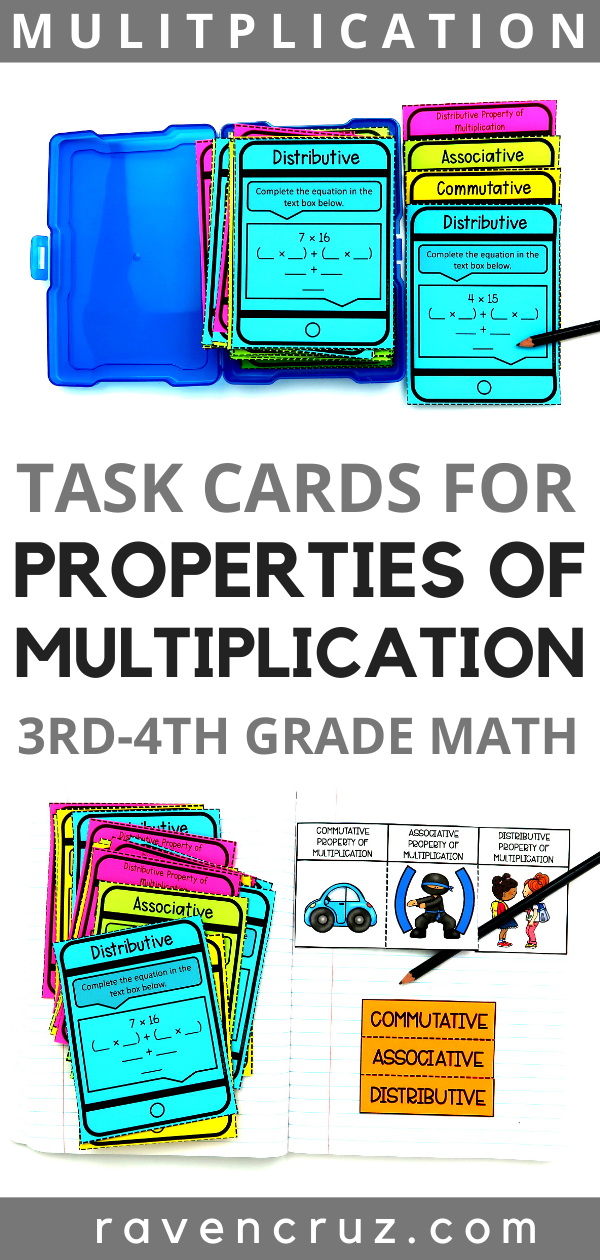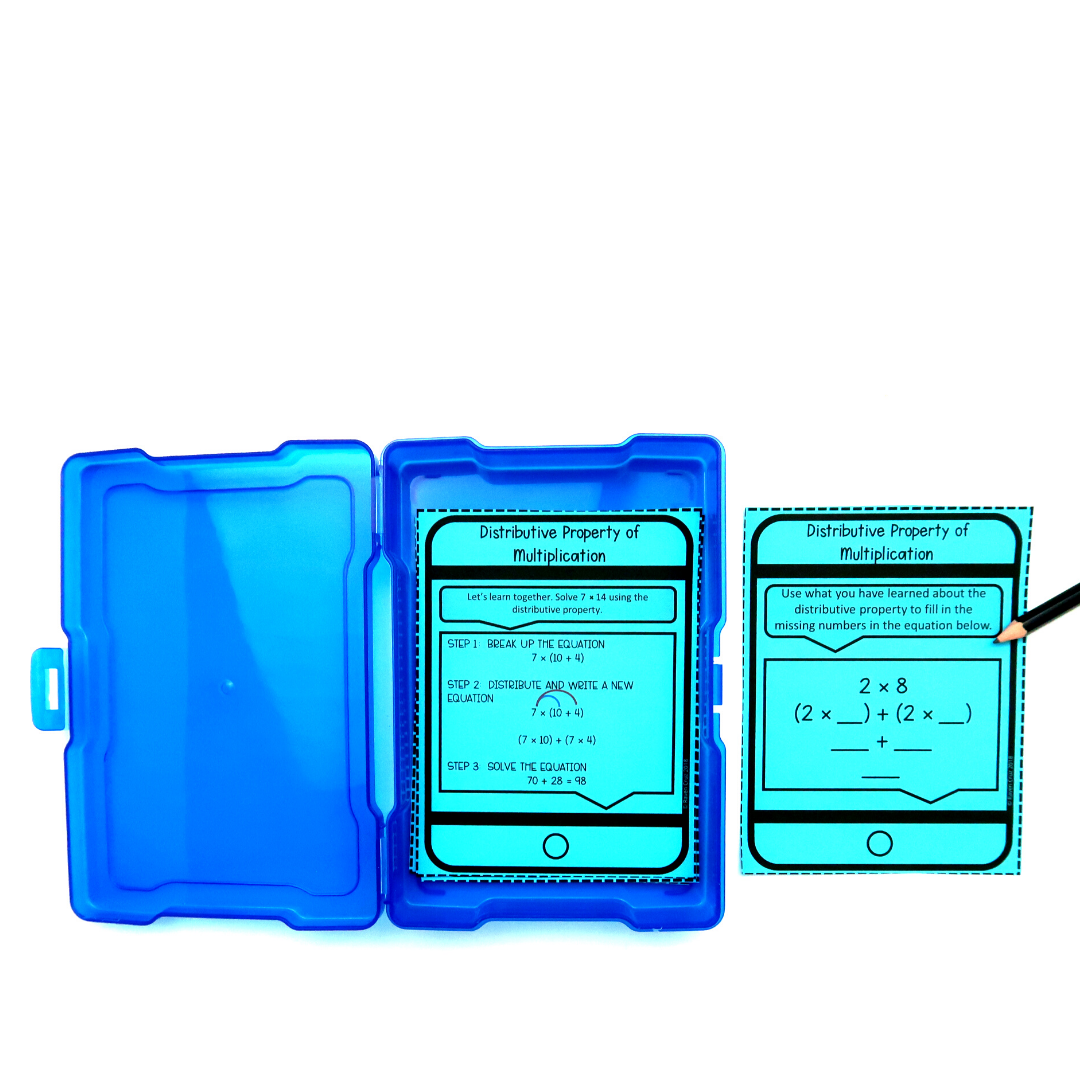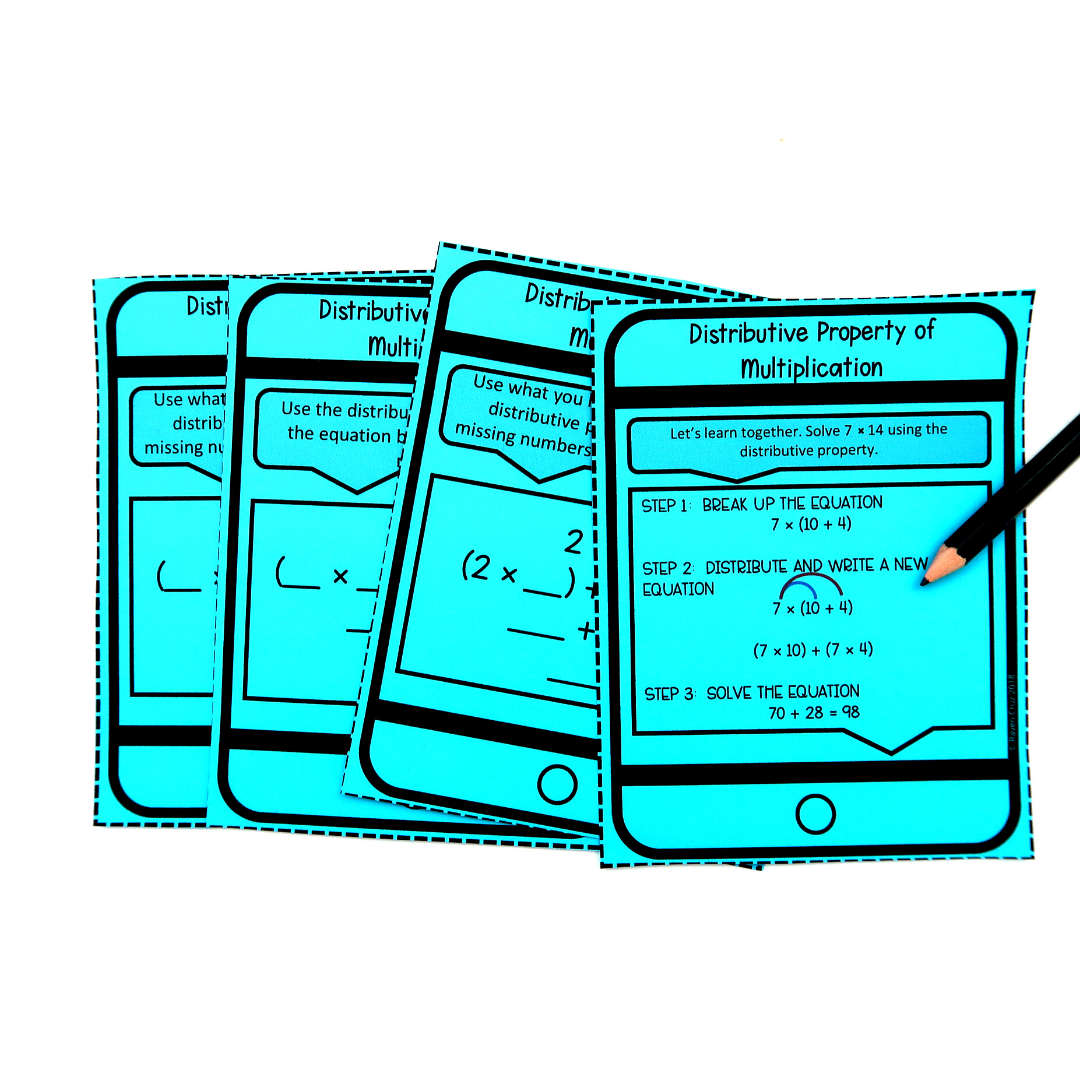Properties of Multiplication Task Cards - Raven Cruz

# Properties of Multiplication Task Cards

What's so great about these properties of multiplication task cards? Personally, I think it is because they come with interactive notebook inserts. Plus, they break down the commutative, associative, and distributive property down for third-grade students to understand.

If you use the task cards for math centers, you can print them on card-stock and laminate. These two steps help with durability and in turn save money because you don't have to reproduce them so often. Alternately, if you don't print them on card-stock, you can always print them on colored paper.

On an opposite note, the properties of multiplication can be a hard task for third-grade students. Third-grade is a hard year (in my professional opinion) for math altogether. Consequently, this is why I worked hard to develop an engaging and useful resource to help reinforce teachers' properties of multiplication curriculum in third-grade.The resource comes with the three required properties: commutative, associative, and distributive, as well as an interactive notebook insert.

## The Commutative Property of Multiplication

The commutative property of multiplication states that the order of the factors (numbers) does not matter the product will be the same.

For example:

5 × 7 = 7 × 5

As you can see, the numbers can move, but the answer will be the same.

## The Associative Property of Multiplication

The associative property of multiplication states that the grouping of a multiplication sentence does not matter, the product will be the same. So in other words, the parenthesis can move on each side of the number sentence, but the numbers do not change places.

For example:

3 × (5 × 7) = (3 × 5) × 7

Notice how the parenthesis are what changes the grouping in the multiplication sentence. The product will in fact be the same, and the numbers DO NOT change places.

## The Distributive Property of Multiplication

The distributive property of multiplication states that when a number is multiplied by the sum of two numbers, the first can be distributed to both of those and multiplied by each separately, then adding the two products together.

First, we "break-up" one of the factors to make it easier for 3rd-graders to multiply. If a student is given the multiplication sentence 8 × 16, it is much easier for the student to "break up" the factor 16

For example:

8 × (10 + 6)

Next, the student will distribute the 8 to the addends and write a new equation.

(8 × 10) + (8 × 6)

Last, solve the equation.

80 + 48 = 128

As you can see, "breaking-up" the factor 16 and distributing the 8 to each addend made the problem much easier to solve. A student does not necessarily have to break 16 up into 10 and 6, but it most cases it is the logical way.

##### WAIT! You may also enjoy the distributive property of multiplication freebie in this blog post